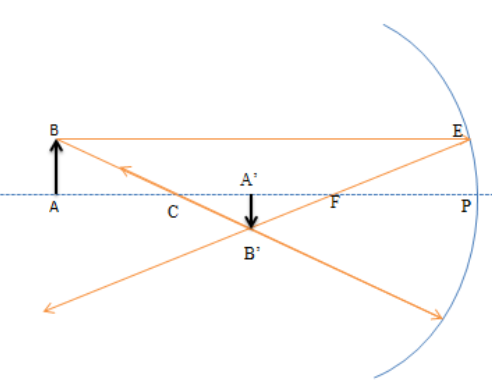QuestionAnswers

# Establish the formula $\dfrac{1}{f} = \dfrac{1}{u} + \dfrac{1}{v}$ for a concave mirror, where symbols have their usual meanings.Hint- A mirror formula can be defined as the formula that gives the relationship between object distance 'u', image distance 'v' and mirror focal length 'f'. The mirror formula is applicable for both plane mirrors and spherical mirrors (convex and concave mirrors).

Complete step-by-step solution -
The mirror formula is written as:
$\dfrac{1}{f} = \dfrac{1}{u} + \dfrac{1}{v}$
Let AB be an object placed on the principal axis of a concave mirror of focal length f. u is the distance between the object and the mirror and v is the distance between the image and the mirror.In the above figure
In $\Delta ABC$ and $\Delta A'B'C$
$\Delta ABC \sim \Delta A'B'C$ (AA similarity rule)
$\dfrac{{AB}}{{A'B'}} = \dfrac{{AC}}{{A'C}}............\left( 1 \right)$
Similarly, in $\Delta FPE$ and $\Delta A'B'F$
$\dfrac{{EP}}{{A'B'}} = \dfrac{{PF}}{{A'F}} \\ \dfrac{{AB}}{{A'B'}} = \dfrac{{PF}}{{A'F}}\left[ {AB = EP} \right].......\left( 2 \right) \\$
From equation (1) and (2)
$\dfrac{{AC}}{{A'C}} = \dfrac{{PF}}{{A'F}} \\ \dfrac{{A'C}}{{AC}} = \dfrac{{A'F}}{{PF}} \\ \dfrac{{\left( {CP - A'P} \right)}}{{\left( {AP - CP} \right)}} = \dfrac{{\left( {A'P - PF} \right)}}{{PF}} \\$
Now, $PF = - f;CP = 2PF = -2f;AP = - u;A'P = - v$
Substituting the above values in the above relation, we will get the answer
$\dfrac{{\left[ {\left( { - 2f} \right) - \left( { - v} \right)} \right]}}{{\left( { - u} \right) - \left( { - 2f} \right)}} = \dfrac{{\left[ {\left( { - v} \right) - \left( { - f} \right)} \right]}}{{\left( { - f} \right)}} \\ uv = fv + uf \\ \dfrac{1}{f} = \dfrac{1}{u} + \dfrac{1}{v} \\$
Thus, the reciprocal of the focal length is numerically equal to the sum of the reciprocals of the distance of the object from the mirror and the distance of the image from the mirror.

Note- Assumptions for Derivation of Mirror Formula:
To obtain the mirror formula the following assumptions are taken. The distances are being measured from the pole of the mirror. According to the convention, the negative sign indicates the distance measured in the direction opposite to the incident ray while the positive sign indicates the distance measured in the direction of the incident ray. The distance below the axis is negative while the above distance is positive.
Physics FormulasFormula for AnglesPhysics Formulas for Class 12 CBSE BoardCBSE Class 10 Math FormulasCBSE Class 12 Physics Nuclei FormulaCBSE Class 12 Physics Atoms FormulaCBSE Class 11 Physics Thermodynamics FormulasCBSE Class 11 Physics Gravitation FormulasTension FormulaSphere FormulaImportant Questions for CBSE Class 12 Physics Chapter 10 - Wave OpticsImportant Questions for CBSE Class 11 Physics Chapter 10 - Mechanical Properties of FluidsImportant Questions for CBSE Class 12 PhysicsImportant Questions for CBSE Class 9 Maths Chapter 12 - Heron's FormulaImportant Questions for CBSE Class 11 PhysicsImportant Questions for CBSE Class 11 Chemistry Chapter 10 - The s-Block ElementsImportant Questions for CBSE Class 6 English Honeysuckle Chapter 10 - The Banyan TreeImportant Questions for CBSE Class 7 English Honeycomb Chapter 10 - The Story of CricketImportant Questions for CBSE Class 8 Science Chapter 10 - Reaching The Age of AdolescenceNCERT Books Free Download for Class 11 Physics Chapter 10 - Mechanical Properties of FluidsCBSE Class 12 Physics Question Paper 2020Previous Year Question Paper for CBSE Class 12 PhysicsPrevious Year Question Paper for CBSE Class 12 Physics - 2015Previous Year Question Paper for CBSE Class 12 Physics - 2018Previous Year Question Paper for CBSE Class 12 Physics - 2014Previous Year Question Paper for CBSE Class 12 Physics - 2013Previous Year Question Paper for CBSE Class 12 Physics - 2019Previous Year Physics Question Paper for CBSE Class 12 - 2017Physics Question Paper for CBSE Class 12 - 2016 Set 1 CMaths Question Paper for CBSE Class 10 - 2012RD Sharma Solutions for Class 9 Maths Chapter 12 - Heron's FormulaNCERT Solutions for Class 9 Maths Chapter 12 Heron’s FormulaLakhmir Singh Physics Class 10 Solutions Chapter 6 - The Human Eyes and The Colorful WorldLakhmir Singh Physics Class 10 SolutionsNCERT Solutions for Class 11 Physics Chapter 10NCERT Solutions for Class 12 Physics Chapter 10Lakhmir Singh Class 10 Solutions for Physics, Chemistry & BiologyLakhmir Singh Physics Class 10 Solutions Chapter 2 - Magnetic Effects of Electric CurrentNCERT Exemplar for Class 11 Physics Chapter 10 - Mechanical Properties of Fluids (Book Solutions)Lakhmir Singh Physics Class 10 Solutions Chapter 5 - Refraction of Light